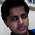### Minimum and Maximum

Source: IITM Friend (who did not want his name to be disclosed). Also read it once in CLR (Introduction to Algorithms)

Given an array of n numbers. Finding minimum takes n-1 comparisons. Finding maximum takes n-1 comparisons. If you had to simultaneously find both minimum and maximum, can you do it in less than 2n-2 comparisons?

Update (11/12/09): Solution by Ameya and Me in comments !!

1.2.first find minimum in n-1 comparision,then find maximum out of n number using n-2 comparision,total of 2n-3 comparision :P

3.itne bure din aa gaye tere.. aisa solution dega tu? your good days are gone man!! you dont rock now!! :P

@rohit..
dude... solution??

4.ya.. u can..
use recursion logic...
and for base case,
when array has length = 2,
u need 1 comparion and not 2 to get min and max. so,
calc(2) = 1,
calc(2^k) = 2* calc(2^(k-1)) + 2
it will solve as
calc(2^k) = 2^(k+1) - 2 - 2^(k-1)

like calc(4) = 4 not 6

calc(8) = 10 not 14

Not if u have array and random length... just apply this logic on first 2^k numbers in it (for max k)... for remaining, use normal approach...
it will definitely have solution < 2n-2

5.@ameya.. fundoo solution

An iterative approach (which is essentially the same) is probably easier to understand:

Break n numbers into pairs of 2.
So, that is n/2 pairs. Find maximum and minimum in each pair. Cost = n/2.
Now given n/2 maximums, find the maximum. Cost = n/2. Given n/2 minimums, find the minimum. Cost = n/2

So, overall cost 3n/2 comparisons. :)

6.One obvious reasoning can be this.
Let say you have parsed till L elements out of N (say, L < N). You have stored a Max and a Min over L elements till now. Now you encounter the (L+1)th. You compare it with Max, If it is greater than or equal to Max, then you don't need to compare it with the Min. So, if you follow this rule throughout the algorithm, you will save at least a computation in (n-1/n) fraction of times you solve this problem. You will have 2n - 2 computations when the first element is actually the maximum in the array, Else you will save. :-)

@pratik at first, I also thought about it the same way as jaadu has written :-D

7.Ameya's solution is more efficient. if N is a power of 2 Ameya's solution uses only N+2 comparisons much less than than 2N-2 or 3/2 N if N is large. If N is not a power of 2. Let k is the largest power such that 2^k <N. write N = 2^k + 2^(k-1) + p . Here p is definitely less than N/4. Now from the first 2^k numbers we get maximum and minimum in 2^k + 2 comparisons. similarly in 2^(k-1) + 2 comparisons we get maximum and minimum of next 2^k-1 numbers and using normal approach we get maximum and minimum of remaining p elements in 2p-2 comparisons. total number of comparisons so far is 2^k +2^(k-1) + 2p +2 = N+p+2. We require 4 more comparisons to get maximum and minimum of whole array. Hence the total number of comparisons is N+p+6 < N+N/4+6 < 3N/2 if N is large.